# Maharashtra Board 10th Class Maths Part 2 Practice Set 1.2 Solutions Chapter 1 Similarity

Balbharti Maharashtra State Board Class 10 Maths Solutions covers the Practice Set 1.2 Geometry 10th Class Maths Part 2 Answers Solutions Chapter 1 Similarity.

## Practice Set 1.2 Geometry10th Std Maths Part 2 Answers Chapter 1 Similarity

Question 1.
Given below are some triangles and lengths of line segments. Identify in which figures, ray PM is the bisector of ∠QPR.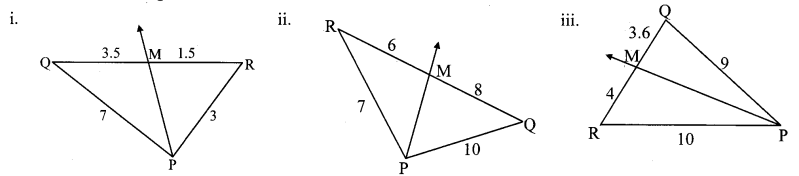Solution:
In ∆ PQR,
$$\frac { PQ }{ PR }$$ = $$\frac { 7 }{ 3 }$$ (i)
$$\frac { QM }{ RM }$$ = $$\frac { 3.5 }{ 1.5 }$$ = $$\frac { 35 }{ 15 }$$ = $$\frac { 7 }{ 3 }$$ (ii)
∴ $$\frac { PQ }{ PR }$$ = $$\frac { QM }{ RM }$$ [From (i) and (ii)]
∴ Ray PM is the bisector of ∠QPR. [Converse of angle bisector theorem]

ii. In ∆PQR,
$$\frac { PQ }{ PR }$$ = $$\frac { 10 }{ 7 }$$ (i)
$$\frac { QM }{ RM }$$ = $$\frac { 8 }{ 6 }$$ = $$\frac { 4 }{ 3 }$$ (ii)
∴ $$\frac { PQ }{ PR }$$ ≠ $$\frac { QM }{ RM }$$ [From (i) and (ii)]
∴ Ray PM is not the bisector of ∠QPR

iii. In ∆PQR,
$$\frac { PQ }{ PR }$$ = $$\frac { 9 }{ 10 }$$ (i)
$$\frac { QM }{ RM }$$ = $$\frac { 3.6 }{ 4 }$$ = $$\frac { 36 }{ 40 }$$ = $$\frac { 9 }{ 10 }$$ (ii)
∴ $$\frac { PQ }{ PR }$$ = $$\frac { QM }{ RM }$$ [From (i) and (ii)]
∴ Ray PM is the bisector of ∠QPR [Converse of angle bisector theorem]

Question 2.
In ∆PQR PM = 15, PQ = 25, PR = 20, NR = 8. State whether line NM is parallel to side RQ. Give reason.Solution:
PN + NR = PR [P – N – R]
∴ PN + 8 = 20
∴ PN = 20 – 8 = 12
Also, PM + MQ = PQ [P – M – Q]
∴ 15 + MQ = 25∴ line NM || side RQ [Converse of basic proportionality theorem]

Question 3.
In ∆MNP, NQ is a bisector of ∠N. If MN = 5, PN = 7, MQ = 2.5, then find QP.Solution:
In ∆MNP, NQ is the bisector of ∠N. [Given]
∴$$\frac { PN }{ MN }$$ = $$\frac { QP }{ MQ }$$ [Property of angle bisector of a triangle]
∴$$\frac { 7 }{ 5 }$$ = $$\frac { QP }{ 2.5 }$$
∴ QP = $$\frac { 7\times 2.5 }{ 5 }$$
∴ QP = 3.5 units

Question 4.
Measures of some angles in the figure are given. Prove that $$\frac { AP }{ PB }$$ = $$\frac { AQ }{ QC }$$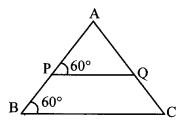Solution:
Proof
∠APQ = ∠ABC = 60° [Given]
∴ ∠APQ ≅ ∠ABC
∴ side PQ || side BC (i) [Corresponding angles test]
In ∆ABC,
sidePQ || sideBC [From (i)]
∴$$\frac { AP }{ PB }$$ = $$\frac { AQ }{ QC }$$ [Basic proportionality theorem]

Question 5.
In trapezium ABCD, side AB || side PQ || side DC, AP = 15, PD = 12, QC = 14, find BQ.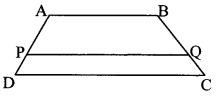Solution:
side AB || side PQ || side DC [Given]
∴$$\frac { AP }{ PD }$$ = $$\frac { BQ }{ QC }$$ [Property of three parallel lines and their transversals]
∴$$\frac { 15 }{ 12 }$$ = $$\frac { BQ }{ 14 }$$
∴ BQ = $$\frac { 15\times 14 }{ 12 }$$
∴ BQ = 17.5 units

Question 6.
Find QP using given information in the figure.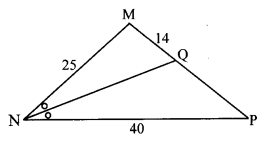Solution:
In ∆MNP, seg NQ bisects ∠N. [Given]
∴$$\frac { PN }{ MN }$$ = $$\frac { QP }{ MQ }$$ [Property of angle bisector of a triangle]
∴$$\frac { 40 }{ 25 }$$ = $$\frac { QP }{ 14 }$$
∴ QP = $$\frac { 40\times 14 }{ 25 }$$
∴ QP = 22.4 units

Question 7.
In the adjoining figure, if AB || CD || FE, then find x and AE.Solution:
line AB || line CD || line FE [Given]
∴$$\frac { BD }{ DF }$$ = $$\frac { AC }{ CE }$$ [Property of three parallel lines and their transversals]
∴$$\frac { 8 }{ 4 }$$ = $$\frac { 12 }{ X }$$
∴ X = $$\frac { 12\times 4 }{ 8 }$$
∴ X = 6 units
Now, AE AC + CE [A – C – E]
= 12 + x
= 12 + 6
= 18 units
∴ x = 6 units and AE = 18 units

Question 8.
In ∆LMN, ray MT bisects ∠LMN. If LM = 6, MN = 10, TN = 8, then find LT.Solution:
In ∆LMN, ray MT bisects ∠LMN. [Given]
∴$$\frac { LM }{ MN }$$ = $$\frac { LT }{ TN }$$ [Property of angle bisector of a triangle]
∴$$\frac { 6 }{ 10 }$$ = $$\frac { LT }{ 8 }$$
∴ LT = $$\frac { 6\times 8 }{ 10 }$$
∴ LT = 4.8 units

Question 9.
In ∆ABC,seg BD bisects ∠ABC. If AB = x,BC x+ 5, AD = x – 2, DC = x + 2, then find the value of x.Solution:
In ∆ABC, seg BD bisects ∠ABC. [Given]
∴$$\frac { AB }{ BC }$$ = $$\frac { AD }{ CD }$$ [Property of angle bisector of a triangle]
∴$$\frac { x }{ x+5 }$$ = $$\frac { x-2 }{ x+2 }$$
∴ x(x + 2) = (x – 2)(x + 5)
∴ x2 + 2x = x2 + 5x – 2x – 10
∴ 2x = 3x – 10
∴ 10 = 3x – 2x
∴ x = 10

Question 10.
In the adjoining figure, X is any point in the interior of triangle. Point X is joined to vertices of triangle. Seg PQ || seg DE, seg QR || seg EF. Fill in the blanks to prove that, seg PR || seg DF.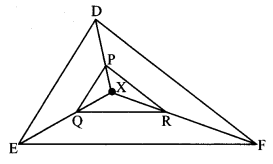Solution: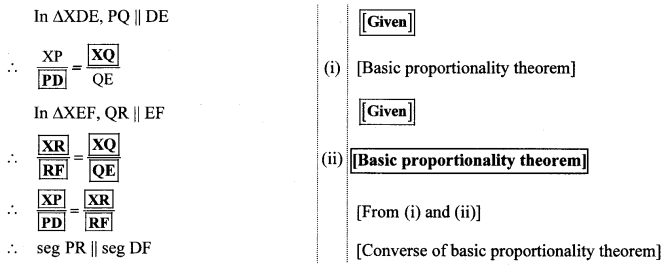Question 11.
In ∆ABC, ray BD bisects ∠ABC and ray CE bisects ∠ACB. If seg AB = seg AC, then prove that ED || BC.Solution:
In ∆ABC, ray BD bisects ∠ABC. [Given]
∴$$\frac { AB }{ BC }$$ = $$\frac { AE }{ EB }$$ (i) [Property of angle bisector of a triangle]
Also, in ∆ABC, ray CE bisects ∠ACB. [Given]
∴$$\frac { AC }{ BC }$$ = $$\frac { AE }{ EB }$$ (ii) [Property of angle bisector of a triangle]
But, seg AB = seg AC (iii) [Given]
∴$$\frac { AB }{ BC }$$ = $$\frac { AE }{ EB }$$ (iv) [From (ii) and (iii)]
∴$$\frac { AD }{ DC }$$ = $$\frac { AE }{ EB }$$ [From (i) and (iv)]
∴ ED || BC [Converse of basic proportionality theorem]

Question 1.
i. Draw a ∆ABC.
ii. Bisect ∠B and name the point of intersection of AC and the angle bisector as D.
iii. Measure the sides.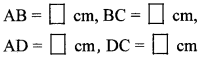iv. Find ratios $$\frac { AB }{ BC }$$ and $$\frac { AD }{ DC }$$
v. You will find that both the ratios are almost equal.
vi. Bisect remaining angles of the triangle and find the ratios as above. Verify that the ratios are equal. (Textbook pg. no. 8)
Solution: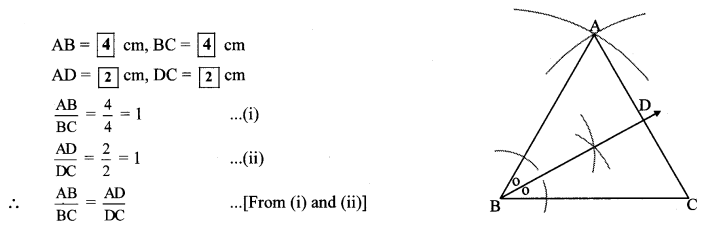Note: Students should bisect the remaining angles and verify that the ratios are equal.

Question 2.
Write another proof of the above theorem (property of an angle bisector of a triangle). Use the following properties and write the proof.
i. The areas of two triangles of equal height are proportional to their bases.
ii. Every point on the bisector of an angle is equidistant from the sides of the angle. (Textbook pg. no. 9)Given: In ∆CAB, ray AD bisects ∠A.
To prove: $$\frac { AB }{ AC }$$ = $$\frac { BD }{ DC }$$
Construction: Draw seg DM ⊥ seg AB A – M – B and seg DN ⊥ seg AC, A – N – C.
Solution:
Proof:
In ∆ABC,
Point D is on angle bisector of ∠A. [Given]
∴DM = DN [Every point on the bisector of an angle is equidistant from the sides of the angle]
$$\frac{A(\Delta A B D)}{A(\Delta A C D)}=\frac{A B \times D M}{A C \times D N}$$ [Ratio of areas of two triangles is equal to the ratio of the product of their bases and corresponding heights]
∴ $$\frac{A(\Delta A B D)}{A(\Delta A C D)}=\frac{A B}{A C}$$ (ii) [From (i)]
Also, ∆ABD and ∆ACD have equal height.
∴ $$\frac{\mathrm{A}(\Delta \mathrm{ABD})}{\mathrm{A}(\Delta \mathrm{ACD})}=\frac{\mathrm{BD}}{\mathrm{CD}}$$ (iii) [Triangles having equal height]
∴$$\frac{\mathrm{AB}}{\mathrm{AC}}=\frac{\mathrm{BD}}{\mathrm{DC}}$$ [From (ii) and (iii)]

Question 3.
i. Draw three parallel lines.
ii. Label them as l, m, n.
iii. Draw transversals t1 and t2.
iv. AB and BC are intercepts on transversal t1.
v. PQ and QR are intercepts on transversal t2.
vi. Find ratios $$\frac { AB }{ BC }$$ and $$\frac { PQ }{ QR }$$. You will find that they are almost equal. Verify that they are equal.(Textbook pg, no. 10)
Solution:(Students should draw figures similar to the ones given and verify the properties.)

Question 4.
In the adjoining figure, AB || CD || EF. If AC = 5.4, CE = 9, BD = 7.5, then find DF.(Textbook pg, no. 12)Solution:Question 5.
In ∆ABC, ray BD bisects ∠ABC. A – D – C, side DE || side BC, A – E – B, then prove that $$\frac { AB }{ BC }$$ = $$\frac { AE }{ EB }$$ (Textbook pg, no. 13)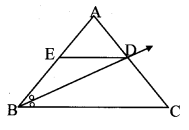Solution: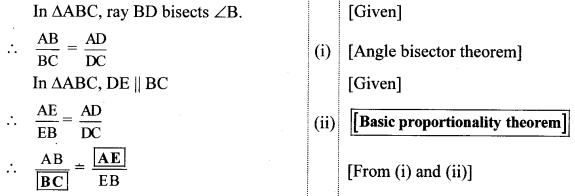Scroll to Top